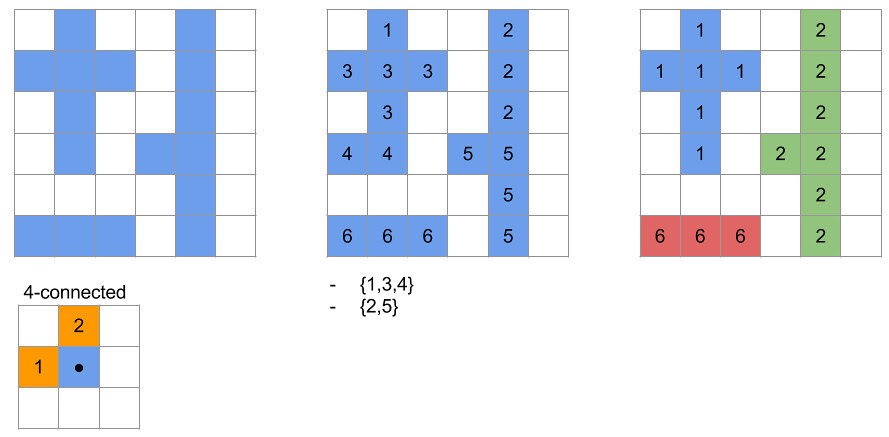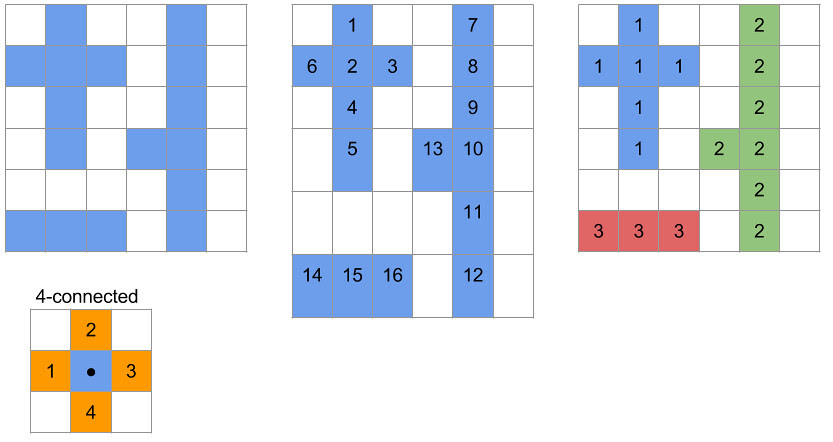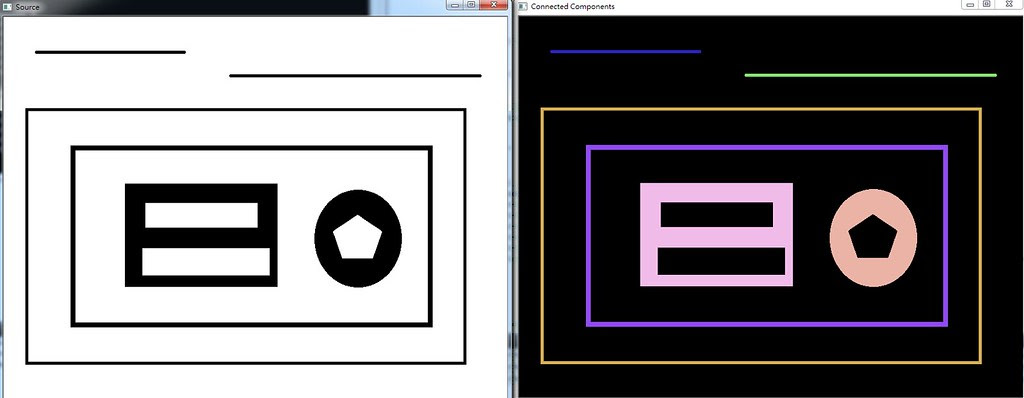# Connected Component Labeling using OpenCV

### Two-Pass Algorithm with 4-connected

• 左方候選像素點(x-1,y)是否已經被賦予標記數字
• 是 - 將候選像素點(x,y)賦予同樣的標記數字，且繼續執行下一個條件檢查
• 否 - 繼續執行下一個條件檢查
• 上方候選像素點(x,y-1)是否已經被賦予標記數字
• 是 - 如果上一個條件有成立，需要執行合併，建立一個群組紀錄此連通物件已被標記哪些數字；如果沒成立，將候選像素點(x,y)賦予同樣的標記數字，且繼續執行下一個條件檢查
• 否 - 繼續執行下一個條件檢查
• 候選像素點(x,y)是否已經被賦予標記數字
• 是 - 繼續執行下一個像素點的檢查
• 否 - 賦予一個新的標記數字

• 候選像素點(x,y)被賦予的標記數字是否為標記群組中數字最小的
• 是 - 繼續檢查下一個像素點
• 否 - 將該像素點的標記數字改成群組中最小的數字代表### Seed Filling Algorithm

• 搜尋方式
• 由左至右，由上至下檢查到尚未標記的候選像素點
• 由候選點開始利用4連通方式搜尋相鄰四個方向的候選點
• 檢查方式
1. 上方候選像素點(x,y-1)是否已被標記且加入連通集合中
• 是 - 繼續執行下一個條件檢查
• 否 - 將候選像素點(x,y)加入該連通集合中，並移動至上方候選像素點(x,y-1)且返回重新檢查的第一步驟
2. 右方候選像素點(x+1,y)是否已被標記且加入連通集合中
• 是 - 繼續執行下一個條件檢查
• 否 - 將候選像素點(x,y)加入該連通集合中，並移動至右方候選像素點(x+1,y)且返回重新檢查的第一步驟
3. 下方候選像素點(x,y+1)是否已被標記且加入連通集合中
• 是 - 繼續執行下一個條件檢查
• 否 - 將候選像素點(x,y)加入該連通集合中，並移動至下方候選像素點(x,y+1)且返回重新檢查的第一步驟
4. 左方候選像素點(x-1,y)是否已被標記且加入連通集合中
• 是 - 移動至上一個位置繼續上為檢查完成的動作
• 否 - 將候選像素點(x,y)加入該連通集合中，並移動至左方候選像素點(x-1,y)且返回重新檢查的第一步驟### `int connectedComponents(InputArray image, OutputArray labels, int connectivity=8, int ltype=CV_32S)`

• `image` 輸入影像
• `labels` 輸出標記完的影像
• `connectivity` 使用4連通或者8連通
• `ltype` 輸出影像的型態 (CV_32S或CV_16U)
``````int threshval = 100;
cv::cvtColor(srcImg, grayImg, cv::COLOR_BGR2GRAY);
cv::Mat binaryImg = threshval < 128 ? (grayImg < threshval) : (grayImg > threshval);
cv::Mat labelImage;
int nLabels = cv::connectedComponents(bw, labelImage, 8);``````

### `int connectedComponentsWithStats(InputArray image, OutputArray labels, OutputArray stats, OutputArray centroids, int connectivity=8, int ltype=CV_32S)`

• `image` 輸入影像
• `labels` 輸出標記完的影像
• `connectivity` 使用4連通或者8連通
• `ltype` 輸出影像的型態 (CV_32S或CV_16U)
• `stats`
• CC_STAT_LEFT 該連通物件最左邊的值(x)
• CC_STAT_TOP 該連通物件最上方的值(y)
• CC_STAT_WIDTH 該連通物件水平的最大寬度(w)
• CC_STAT_HEIGHT 該連通物垂直最大長度(h)
• CC_STAT_AREA 該連通物件的面積
• `centroids` 輸出連通物件的中心點
``````int threshval = 100;
cv::cvtColor(srcImg, grayImg, cv::COLOR_BGR2GRAY);
cv::Mat binaryImg = threshval < 128 ? (grayImg < threshval) : (grayImg > threshval);
cv::Mat labelImage;
int nLabels = cv::connectedComponentsWithStats(binaryImg, labelImage, stats, centroids, 8, CV_32S);

std::cout << "Number of connected components = " << nLabels << std::endl << std::endl;

for(int label = 1; label < nLabels; ++label){
std::cout << "Component "<< label << std::endl;
std::cout << "CC_STAT_LEFT   = " << stats.at<int>(label,cv::CC_STAT_LEFT) << std::endl;
std::cout << "CC_STAT_TOP    = " << stats.at<int>(label,cv::CC_STAT_TOP) << std::endl;
std::cout << "CC_STAT_WIDTH  = " << stats.at<int>(label,cv::CC_STAT_WIDTH) << std::endl;
std::cout << "CC_STAT_HEIGHT = " << stats.at<int>(label,cv::CC_STAT_HEIGHT) << std::endl;
std::cout << "CC_STAT_AREA   = " << stats.at<int>(label,cv::CC_STAT_AREA) << std::endl;
std::cout << "CENTER   = (" << centroids.at<double>(label, 0) <<","<< centroids.at<double>(label, 1) << ")"<< std::endl << std::endl;
}
``````